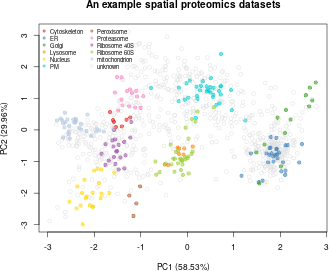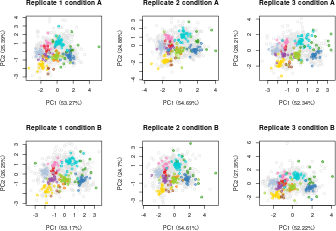``````# options(width = 150)
library("bandle")
library("ggplot2")
library("pRoloc")
library("pRolocdata")
library("MSnbase")
library("pheatmap")
library("viridis")

setStockcol(paste0(getStockcol(), "90")) # settransparent colours``````

# 1 Introduction

Bayesian ANalysis of Differential Localisation Experiments (BANDLE) is an integrative semi-supervised functional mixture model, developed by (Crook et al. 2021), to obtain the probability of a protein being differentially localised between two conditions.

In this vignette we walk users through how to install and use the R (R Development Core Team 2011) Bioconductor (Gentleman et al. 2004) `bandle` package by simulating a well-defined differential localisation experiment from spatial proteomics data from the `pRolocdata` package (Gatto et al. 2014).

The BANDLE method uses posterior Bayesian computations performed using Markov-chain Monte-Carlo (MCMC) and thus uncertainty estimates are available (Gilks, Richardson, and Spiegelhalter 1995). Throughout this vignette we use the term differentially localised to pertain to proteins which are assigned to different sub-cellular localisations between two conditions. One of the main outputs of BANDLE is the probability that a protein is differentially localised between two conditions.

# 2 Installation

The package can be installed with the `BiocManager` package:

``````if (!requireNamespace("BiocManager", quietly=TRUE))
install.packages("BiocManager")
BiocManager::install("bandle")``````

# 3 The data

In this vignette and Crook et al. (2021), the main data source that we use to study differential protein sub-cellular localisation are data from high-throughput mass spectrometry-based experiments. The data from these types of experiments traditionally yield a matrix of measurements wherein we have, for example, PSMs, peptides or proteins along the rows, and samples/channels/fractions along the columns. The `bandle` package uses the `MSnSet` class as implemented in the Bioconductor MSnbase package and thus requires users to import and store their data as a `MSnSet` instance. For more details on how to create a `MSnSet` see the relevant vignettes in pRoloc. The pRolocdata experiment data package is a good starting place to look for test data. This data package contains tens of quantitative proteomics experiments, stored as `MSnSet`s.

## 3.1 A well-defined theoretical example

To get started with the basics of using `bandle` we begin by generating a simple example dataset which simulates a differential localisation experiment (please see the second vignette in this package for a full real-life biological use case). In this example data, the key elements are replicates, and a perturbation of interest. There is code within the bandle package to simulate an example experiment.

In the code chunk below we begin by loading the pRolocdata package to obtain a spatial proteomics dataset. This will be the basis of our simulation which will use boostrapping to generate new datasets. The dataset we have chosen to load is a dataset from 2009 (`tan2009r1`). This is data from a early LOPIT experiment performed on Drosophila embryos (Tan et al. 2009). The aim of this experiment was to apply LOPIT to an organism with heterogeneous cell types. This experiment used four isotopes across four distinct fractions and thus yielded four measurements (features) per protein profile. We visualise the data by using principal components analysis.

``````library("pRolocdata")
data("tan2009r1")
plot2D(tan2009r1,
main = "An example spatial proteomics datasets",
grid = FALSE)
addLegend(tan2009r1, where = "topleft", cex = 0.7, ncol = 2)``````The following code chuck simulates a differential localisation experiment. It will generate `numRep/2` of each a control and treatment condition. We will also simulate relocalisations for `numDyn` proteins.

``````set.seed(1)
tansim <- sim_dynamic(object = tan2009r1,
numRep = 6L,
numDyn = 100L)``````

The list of the 6 simulated experiments are found in `tansim\$lopitrep`. Each one is an `MSnSet` instance (the standard data container for proteomics experimental data). The first 3 are the simulated control experiments (see `tansim\$lopitrep[1:3]`), and the following 3 in the list are the treatment condition simulated experiments (see `tansim\$lopitrep[4:6]`). We can plot them using the `plot2D` function from `pRoloc`.

``````plot_title <- c(paste0("Replicate ", seq(3), " condition", " A"),
paste0("Replicate ", seq(3), " condition", " B"))

par(mfrow = c(2, 3))
out <- lapply(seq(tansim\$lopitrep), function(z)
plot2D(tansim\$lopitrep[[z]], grid = FALSE, main = plot_title[z]))``````For understanding, exploring and visualizing individual spatial proteomics experiments, see the vignettes in `pRoloc` and `MSnbase` packages.

``tansim\$lopitrep[]``
``````## MSnSet (storageMode: lockedEnvironment)
## assayData: 888 features, 4 samples
##   element names: exprs
## protocolData: none
## phenoData
##   sampleNames: X114 X115 X116 X117
##   varLabels: Fractions
## featureData
##   featureNames: P20353 P53501 ... P07909 (888 total)
##   fvarLabels: FBgn Protein.ID ... knn.scores (18 total)
## experimentData: use 'experimentData(object)'
##   pubMedIds: 19317464
## Annotation:
## - - - Processing information - - -
## Added markers from  'mrk' marker vector. Thu Jul 16 22:53:44 2015
## Performed knn prediction (k=10) Tue Apr 26 16:02:42 2022
##  MSnbase version: 1.17.12``````

# 4 Preparing for `bandle` analysis

The main function of the package is `bandle`, this uses a complex model to analyse the data. Markov-Chain Monte-Carlo (MCMC) is used to sample the posterior distribution of parameters and latent variables. From which statistics of interest can be computed. Here we only run a few iterations for brevity but typically one needs to run thousands of iterations to ensure convergence, as well as multiple parallel chains.

## 4.1 Fitting Gaussian processes

First, we need to fit non-parametric regression functions to the markers profiles, upon which we place our analysis. This uses Gaussian processes. The `fitGPmaternPC` function can be used and fits some default penalised complexity priors (see `?fitGP`), which works well. However, these can be altered, which is demonstrated in the next code chunk

``````par(mfrow = c(4, 3))
gpParams <- lapply(tansim\$lopitrep, function(x) fitGPmaternPC(x))``````

We apply the `fitGPmaternPC` function to each datasets by calling `lapply` over the `tansim\$lopitrep` list of datasets. The output of `fitGPmaternPC` returns a list of posterior predictive means and standard deviations. As well as MAP hyperparamters for the GP.

The `plotGPmatern` function can be used to plot the profiles for each class in each replicate condition with the posterior predictive distributions are overlayed with markers protein profiles.

For example, to plot the predictive distributions of the first dataset,

``````par(mfrow = c(4, 3))
plotGPmatern(tansim\$lopitrep[], params = gpParams[])``````